# Rock, Paper, Scissor game – Python Project

• Difficulty Level : Easy
• Last Updated : 08 Aug, 2021

In this article, we will see how we can create a rock paper and scissor game using Tkinter. Rock paper scissor is a hand game usually played between two people, in which each player simultaneously forms one of the three shapes with an outstretched hand. These shapes are “rock”, “paper”, and “scissors”.

## Game Winner Conditions

Let there be a Player who is playing with a computer as an opponent.  Now,

Attention geek! Strengthen your foundations with the Python Programming Foundation Course and learn the basics.

To begin with, your interview preparations Enhance your Data Structures concepts with the Python DS Course. And to begin with your Machine Learning Journey, join the Machine Learning - Basic Level Course

• If the player selects Paper and Computer Selects Scissor – Computer wins
• If the player selects Rock and Computer Selects Scissor – Player 1 wins
• If the player selects Paper and Computer Selects Rock – Player 1 wins
• And If the player selects Paper and Computer Selects Paper – Draw
• If the player selects Rock and Computer Selects Rock – Draw
• If the player selects Scissor and Computer Selects Scissor – Draw

## Tools and Technologies Used

• Tkinter: It is a standard Python interface to the Tk GUI toolkit shipped with Python. Python with tkinter is the fastest and easiest way to create the GUI applications.
• Random: Python defines a set of functions that are used to generate or manipulate random numbers through the random module.
• PIL: Python Imaging Library (expansion of PIL) is the de facto image processing package for Python language. It incorporates lightweight image processing tools that aids in editing, creating and saving images.

## GUI Implementation

### Part 1:Image processing and handling

1) Import Python Module Tkinter to execute GUI applications.

2) From PIL Import ImageTk, Image for image processing.

3) Import random which will help the computer to select options rock, paper, or scissor randomly.

4) Now an object is created root which is the main window object.

• Title of this window -> Rock Paper Scissor
• Dimensions of this window -> “800×680”

5) Create canvas of width=800, height=680

6) Now we Create labels on GUI window

• l1 =Player-> font=’Algerian’, size=25
• l2 =Computer-> font=’Algerian’, size=25
• l3 =Vs font=’Algerian’, size=40

7) Now labels are placed on window

• l1 at x=80, y=20
• l2 at x=560, y=20
• l3 at x=370, y=230

For Default Image:

1) An variable named img_p is used to open the default hand image and resize it to (300,300). This will be shown at default condition in the game at the place of the player.

2) An variable named img_c is used to store flipped default image from left to right using transpose function and it is saved in the variable. This will be shown at default condition in the game at the place of the computer’s side.

3) Images img_p and img_c are loaded on the canvas now using Tk.PhotoImage

For Rock Image:

1) An variable named rock_p is used to open the rock hand image and resize it to (300,300). This will be shown at the player’s side when the player selects rock in the game.

2) An variable named rock_c is used to store flipped rock hand image from left to right using transpose function and will be shown at computer’s side when the computer randomly selects rock in the game.

3) Images rock_p and  rock_c are loaded on the canvas now using Tk.PhotoImage.

For Paper Image

1) An variable named paper_p is used to open the paper hand image and resize it to (300,300). This will be shown at the player’s side when the player selects paper in the game.

2) An variable named paper_c is used to store flipped paper hand images from left to right using the transpose function and will be shown at the computer’s side when the computer randomly selects paper in the game.

3) Images paper_p and  paper_c is loaded on the canvas now using Tk.PhotoImage.

For Scissors Image

1) An variable named scissor_p is used to open the scissor hand image and resize it to (300,300). This will be shown at player’s side when player selects scissor in the game.

2) An variable named scissor_c is used to store flipped scissor hand image from left to right using transpose function and will be shown at computer’s side when computer randomly selects scissor in the game.

3) Images scissor_p and  scissor_c are loaded on the canvas now using Tk.PhotoImage.

For Selection Image:

1) An variable named img_s is used to open the selection of hand images i.e. Combined image of Rock, Paper & Scissor, and resize it to (300,130).

2) Image img_s is loaded on the canvas now using Tk.PhotoImage.

### Part 2: Game Implementation

1) A game function is defined in which we have a list named select having values 1, 2, 3 representing rock, paper, and scissors respectively.

2) Here random.choice randomly selects options 1-3 for computer

3) Set image for Player on canvas

• If Player selects 1 (Rock)  Show rock image on canvas using create_image.
• If Player selects 2 (Paper) Show paper image on canvas using create_image.
• If Player selects 3 (Scissor) Show scissor image on canvas using create_image.

4) Set image for Computer on canvas

• If Computer selects 1 (Rock)  Show rock image on canvas using create_image.
• If Computer selects 2 (Paper) Show paper image on canvas using create_image.
• If Computer selects 3 (Scissor) Show scissor image on canvas using create_image.

5) Obtaining the result

• If Player chooses Rock and computer chooses Rock OR If the player chooses Paper and the computer chooses Paper OR If the player chooses Scissor and the computer chooses Scissor. Result Shown-> Draw
• If the Player chooses Rock and computer choose Scissor OR If the player chooses Paper and computer choose Rock OR If the player chooses Scissor and computer choose Paper.  Result Shown -> Player won
• Else Result Shown-> Computer won

Note: This result is shown on canvas in form of text having dimensions (390,600), font=’Algerian’, tag=’result’.

6) Buttons

• Clear Button -> Deletes the present result and switches the figures at both sides to its default conditions.
• Rock  Button -> Selects choice 1 in function game and Shows an Image of Hand showing Rock On Player side.
• Paper Button -> Selects choice 2 in function game and Shows Image of Hand showing Paper On Player side
• Scissor Button -> Selects choice 3 in function game and Shows an Image of Hand showing the Scissor On the Player side.

Images Used: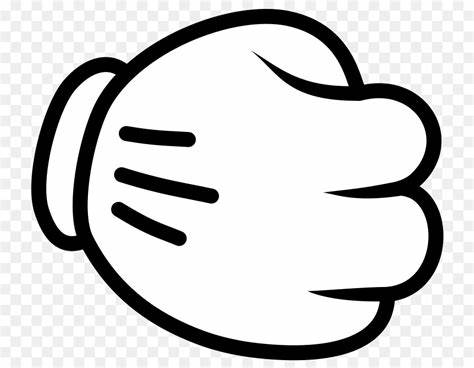default.jpg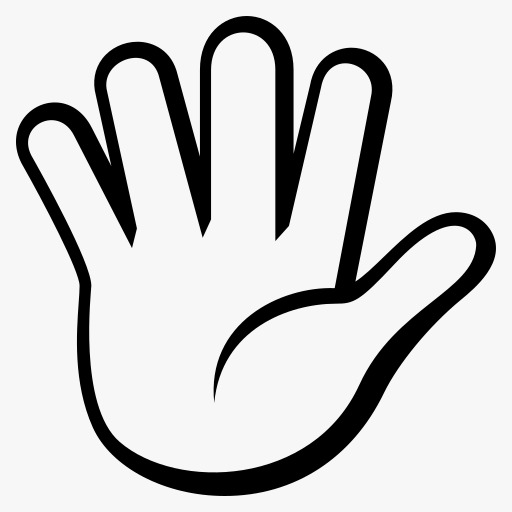paper.jpg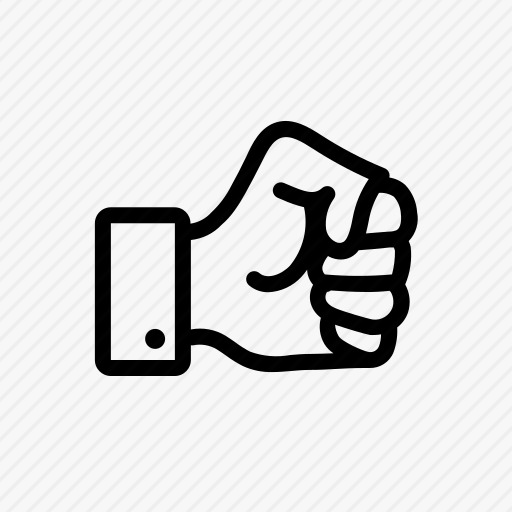rock.jpg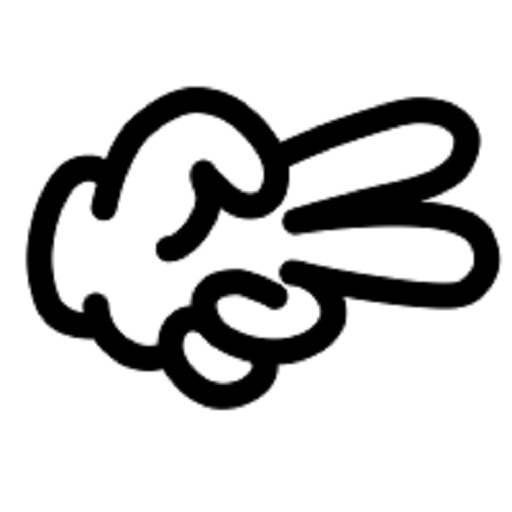scissor.jpg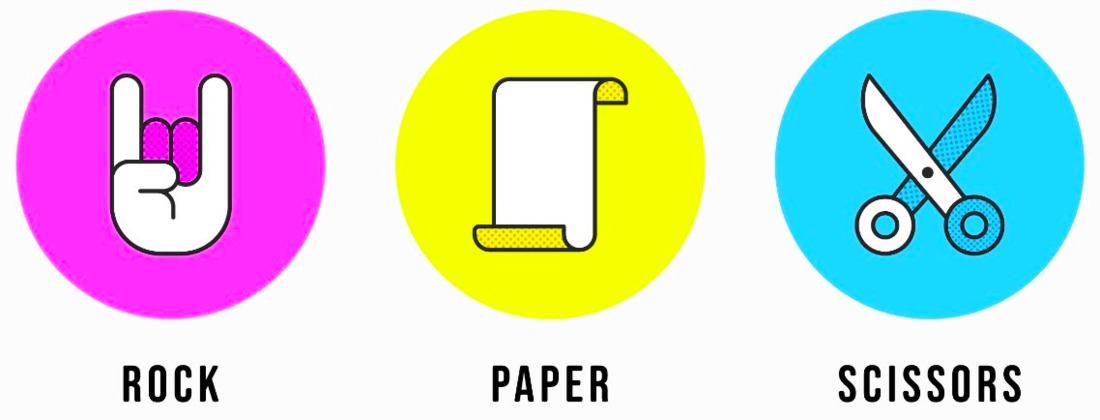Selection.jpg

main.py

## Python3

 `from` `tkinter ``import` `*``from` `PIL ``import` `ImageTk, Image``import` `random` `# main window object``root ``=` `Tk()` `# Title of GUI window``root.title(``'Rock Paper Scissor'``)` `# Size of window``root.geometry(``'800x680'``)` `# Creating canvas``canvas ``=` `Canvas(root, width``=``800``, height``=``680``)``canvas.grid(row``=``0``, column``=``0``)` `# Creating labels on GUI window``l1 ``=` `Label(root, text``=``'Player'``, font``=``(``'Algerian'``, ``25``))``l2 ``=` `Label(root, text``=``'Computer'``, font``=``(``'Algerian'``, ``25``))``l3 ``=` `Label(root, text``=``'Vs'``, font``=``(``'Algerian'``, ``40``))` `# Placing all the labels on window``l1.place(x``=``80``, y``=``20``)``l2.place(x``=``560``, y``=``20``)``l3.place(x``=``370``, y``=``230``)` `# Default image``img_p ``=` `Image.``open``(``"default.jpeg"``)``img_p ``=` `img_p.resize((``300``, ``300``))` `# Flipping image from left to right``img_c ``=` `img_p.transpose(Image.FLIP_LEFT_RIGHT)` `# Loading images to put on canvas``img_p ``=` `ImageTk.PhotoImage(img_p)``img_c ``=` `ImageTk.PhotoImage(img_c)` `# Rock image``rock_p ``=` `Image.``open``(``'rock.jpeg'``)``rock_p ``=` `rock_p.resize((``300``, ``300``))` `# Flipping image from left to right``rock_c ``=` `rock_p.transpose(Image.FLIP_LEFT_RIGHT)` `# Loading images to put on canvas``rock_p ``=` `ImageTk.PhotoImage(rock_p)``rock_c ``=` `ImageTk.PhotoImage(rock_c)` `# Paper image``paper_p ``=` `Image.``open``(``'paper.jpeg'``)``paper_p ``=` `paper_p.resize((``300``, ``300``))` `# Flipping image from left to right``paper_c ``=` `paper_p.transpose(Image.FLIP_LEFT_RIGHT)` `# Loading images to put on canvas``paper_p ``=` `ImageTk.PhotoImage(paper_p)``paper_c ``=` `ImageTk.PhotoImage(paper_c)` `# Scissor image``scissor_p ``=` `Image.``open``(``'scissor.jpeg'``)``scissor_p ``=` `scissor_p.resize((``300``, ``300``))` `# Flipping image from left to right``scissor_c ``=` `scissor_p.transpose(Image.FLIP_LEFT_RIGHT)` `# Loading images to put on canvas``scissor_p ``=` `ImageTk.PhotoImage(scissor_p)``scissor_c ``=` `ImageTk.PhotoImage(scissor_c)` `# Selection image``img_s ``=` `Image.``open``(``"Selection.jpeg"``)``img_s ``=` `img_s.resize((``300``, ``130``))``img_s ``=` `ImageTk.PhotoImage(img_s)` `# Putting image on canvas on specific coordinates``canvas.create_image(``0``, ``100``, anchor``=``NW, image``=``img_p)``canvas.create_image(``500``, ``100``, anchor``=``NW, image``=``img_c)``canvas.create_image(``0``, ``400``, anchor``=``NW, image``=``img_s)``canvas.create_image(``500``, ``400``, anchor``=``NW, image``=``img_s)`  `# game function``def` `game(player):``    ``select ``=` `[``1``, ``2``, ``3``]``    ` `    ``# Randomly selects option for computer``    ``computer ``=` `random.choice(select)` `    ``# Setting image for player on canvas``    ``if` `player ``=``=` `1``:``      ` `        ``# Puts rock image on canvas``        ``canvas.create_image(``0``, ``100``, anchor``=``NW, image``=``rock_p)``    ``elif` `player ``=``=` `2``:``        ` `        ``# Puts paper image on canvas``        ``canvas.create_image(``0``, ``100``, anchor``=``NW, image``=``paper_p)``    ``else``:``        ` `        ``# Puts scissor image on canvas``        ``canvas.create_image(``0``, ``100``, anchor``=``NW, image``=``scissor_p)` `    ``# Setting image for computer on canvas``    ``if` `computer ``=``=` `1``:``        ` `        ``# Puts rock image on canvas``        ``canvas.create_image(``500``, ``100``, anchor``=``NW, image``=``rock_c)``    ``elif` `computer ``=``=` `2``:``        ` `        ``# Puts paper image on canvas``        ``canvas.create_image(``500``, ``100``, anchor``=``NW, image``=``paper_c)``    ``else``:``        ` `        ``# Puts scissor image on canvas``        ``canvas.create_image(``500``, ``100``, anchor``=``NW, image``=``scissor_c)` `    ``# Obtaining result by comparison``    ``if` `player ``=``=` `computer:  ``# Case of DRAW``        ``res ``=` `'Draw'``        ` `    ``# Case of player's win``    ``elif` `(player ``=``=` `1` `and` `computer ``=``=` `3``) ``or` `(player ``=``=` `2` `and` `computer ``=``=` `1``) ``or``    ``(player ``=``=` `3` `and` `computer ``=``=` `2``):``        ``res ``=` `'You won'``    ` `    ``# Case of computer's win``    ``else``:``        ``res ``=` `'Computer won'` `    ``# Putting result on canvas``    ``canvas.create_text(``390``, ``600``, text``=``'Result:- '` `+` `res,``                       ``fill``=``"black"``, font``=``(``'Algerian'``, ``25``), tag``=``'result'``)`  `# Function for clear button``def` `clear():``  ` `    ``# Removes result from canvas``    ``canvas.delete(``'result'``)` `    ``# Puts default image on canvas``    ``canvas.create_image(``0``, ``100``, anchor``=``NW, image``=``img_p)``    ``canvas.create_image(``500``, ``100``, anchor``=``NW, image``=``img_c)`  `# Button for selecting rock``rock_b ``=` `Button(root, text``=``'Rock'``, command``=``lambda``: game(``1``))``rock_b.place(x``=``35``, y``=``487``)` `# Button for selecting paper``paper_b ``=` `Button(root, text``=``'Paper'``, command``=``lambda``: game(``2``))``paper_b.place(x``=``128``, y``=``487``)` `# Button for selecting scissor``scissor_b ``=` `Button(root, text``=``'Scissor'``, command``=``lambda``: game(``3``))``scissor_b.place(x``=``220``, y``=``487``)` `# Button for clear``clear_b ``=` `Button(root, text``=``'CLEAR'``, font``=``(``'Times'``, ``10``, ``'bold'``),``                 ``width``=``10``, command``=``clear).place(x``=``370``, y``=``28``)` `root.mainloop()`

Output

### Team Members

My Personal Notes arrow_drop_up